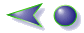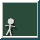Author: Ton Lecluse

Probability tree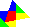IntroductionThe construction boxPlane geometryInvestigating a sectionInvestigating a functionDrawing in perspectiveA probability treeThe calculatorGetting a tasteDownloadsRegisterBack to start

This exercise requires you to set the difficulty level to high (in the configuration window). Geocadabra offers a few nice applications when it comes to probability. This demo allows for a limited probability tree to be drawn.

We are about to solve the following problem.

A vase is filled with 2 red and 8 blue balls.
After you have paid € 1,25 you are allowed to pick two balls in one go. Eyes closed of course!You get paid € 3,- for every red ball and nothing for every blue ball you pick. Do you expect your chances of winning to increase or decrease depending on the number of turns?

Analysis of the model.

What we are dealing with is a draw without replacement from a vase containing 2 red and 8 blue balls.

A probability tree will help us list all possible draws in one chart. By processing this probability tree and by determining the expected value of the turn out you get an impression of whether you will get paid more or less than your original stake.

 A computation using Geocadabra. We will start by selecting a new drawing. Choose statistical methods, probability tree and press [OK]. A probability tree box appears in which we can now select the data we want to use.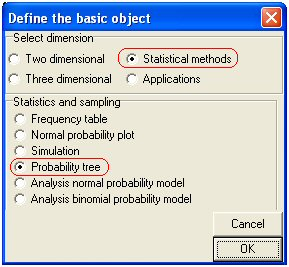Mind the details:The sampling type should be without
replacementName of knot points: numerical.
After all, we wish to calculate using
amounts.Depth: 2;
We will take 2 balls from the vaseSplit count per knot: 2.
You can either pick a blue or a red
ball so there are 2 possibilities per
ballProcess knot points: sum;
Every red ball is linked to € 3,- and
every blue ball to € 0,- and it is
these amounts you wish to
calculate with.Numerical information at each
branch: probability (as a fraction)
Starting values of each type:
2 (red) and 8 (blue).Value of each type: € 3,- for the red ball and € 0,- for the blue ballYou can click on the red or the blue square to select a colour. In this example the colours have already
been set.

Now click the [process] button. The tree is drawn.

Sometimes the texts in the graph right from the tree are off screen. Click the buttons where it says “tree measurements” to adjust the size of the tree horizontally or vertically.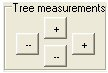The tree will look like below:

If you were to, for example, follow the top branch you would get the following information:The probability of getting two red balls equals. The sum of the total turnout (€ 3,- per red ball) equals € 6,-.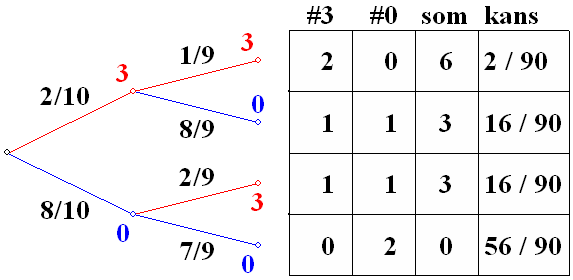Now that the tree has been drawn and processed a new buttonhas appeared. Click it and a box will appear reading the continuation computations. Now select:Variable: sum After all, you would like to know the way the fraction functionsShow results as a fraction: select You would rather not deal with decimals.  The bottom three lines show the sum’s probability distributions. The bottom line tells you that the chance to receive a € 6,- turnout equals. This is theof the probability tree.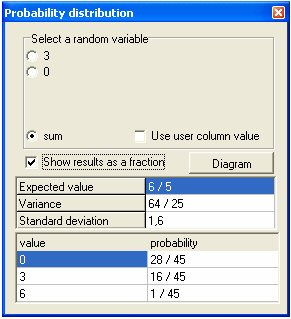The probability of receiving € 3,- equals. This is the sum of the turnouts of the two branches that both generate € 3,-. You can now start interpreting this probability distribution:Out of every 45 times you enter the draw you will once receive € 6,-, 16 times € 3,- and 28 times you will receive nothing at all. This comes down toeuro a turn so that is an average of € 1,20. Since you have to pay € 1,25 to enter the draw you will eventually loose € 0,05 every time you enter. Epilogue Geocadabra can calculate models of probability that can be simulated by means of a draw from a vase with or without replacement. The number of balls that can be drawn is adjustable and the same applies to individual colours. However, the maximum of adjustable colours is limited to 10. Instead of working with colours you can also work with related figures as is shown in the turnout above. It is also possible to work with variables (or stochastic models) per colour or you can work with the sum, the product or even with the maximum or minimum of the drawn numbers. You can save a model of probability to continue working on it at a later date. The probability distribution screen has a button called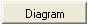Pressing this will result in a histogram (= vertical bar graph) of the current probability distribution and this graph can then be further investigated. You can use a boxplot or you can use the normal distribution (including expected value and standard deviation both equal to those of the probability distribution). In return, you can use these to investigate whether a vase model more or less meets the characteristics of a normally distributed model. You can even have the probability distribution drawn out on regular probability plotting paper. According to the theory this should result in a straight line when using an exact normal model. You can now use the rules of thumb as they apply to a normally distributed model. Alongside is an impression of the model above but things do not start becoming truly interesting until you start with a model that has more depth (and colours). This program will help you support a wonderful learning curve right to the final levels of secondary education.